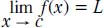NextPrevious

# What is a limit in calculus?

A limit is a fundamental concept in calculus. Unlike a limit mentioned above (as in a series or sequence), a limit of a function in calculus takes on a somewhat different meaning. In particular, a limit of a function can be described as the following: If f(x) is a function defined around a point c (but may not be at c itself), the formal limit equation becomes:Thus, the number L is called the limit of f(x) when x goes to c.

Close

This is a web preview of the "The Handy Math Answer Book" app. Many features only work on your mobile device. If you like what you see, we hope you will consider buying. Get the App